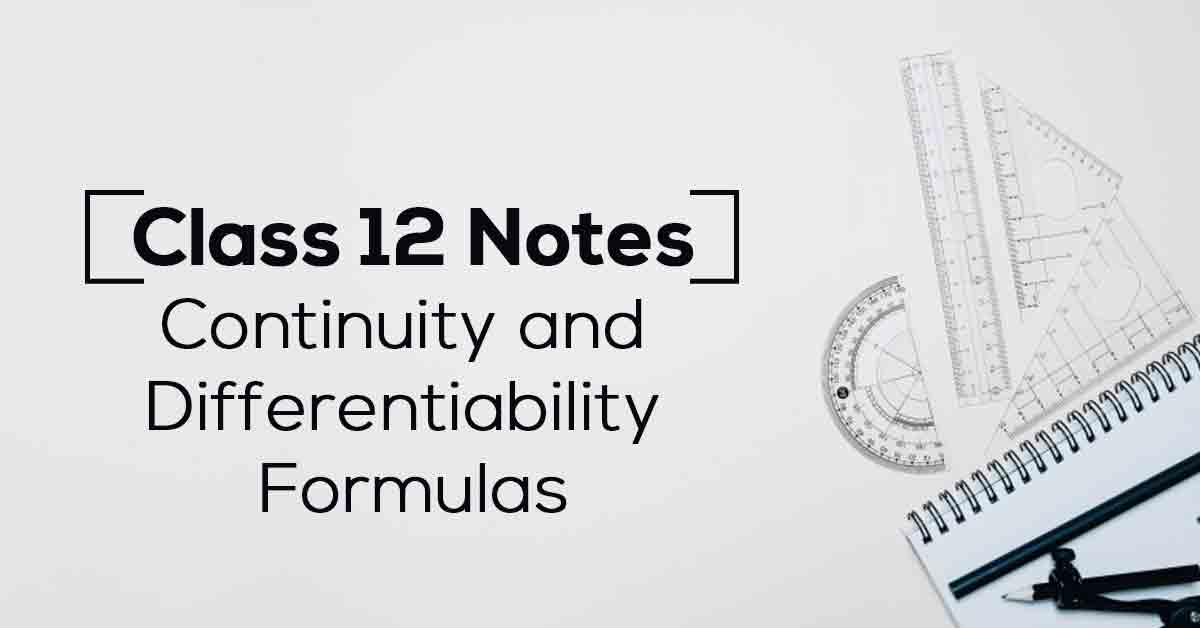Class 12th Math Continuity and Differentiability Formulas CBSE 2023

# Continuity and Differentiability Class 12 Notes# Chapter 5 Continuity and Differentiability Formulas

Students must thoroughly solve the 5th chapter of NCERT Class 12 Maths, ‘Continuity and Differentiability’, to excel in Class 12 board exams. NCERT notes Class 12 Maths Chapter 5 is a valuable resource that helps students grasp the step-by-step approach for solving problems, leading to scoring better marks.

Class 12 Maths Chapter 5 talks about the Algebra of Continuous Functions, Differentiability, Derivatives of Composite and Implicit Functions, Exponential and Logarithmic Functions, Second Order Derivatives, and many more. Keep reading to access the NCERT notes for Maths Class 12 Chapter 5.

### Points to Remember

Below mentioned are some of the important points to be remembered from NCERT Class 12 Maths Chapter 5:

• Following functions are everywhere continuous:
(a) A constant function
(b) The identity function
(c) A polynomial function
(d) Modulus function
(e) Exponential function
(f) Sine and Cosine functions

• Following functions are continuous in their domains:
a) A logarithmic function
(b) A rational function
(c) Tangent, cotangent, secant and cosecant functions
(d) All inverse trigonometric functions are continuous in their respective domains

• A function f(x) is said to be continuous if it is continuous at every point on its domain.

• Rolle’s Theorem:
Let f be a real value of function defined on the closed interval [a, b] such that
(i) It is continuous on [a,b]
(ii) It is differentiable on (a,b) and
(iii) f(a)=f(b)
Then, there exists at least one real number c∈(a,b) such that f'(c)=0.

• Lagrange’s Mean Value Theorem:
Let f(x) be a function defined on [a, b] such that
(i) It is continuous on [a,b] and
(ii) Differentiable on (a,b)
Then, there exists at least one c∈(a,b) such that f'(c)=f(b)-f(a)/b-a

### Topics and Sub-topics

Continuity and Differentiability may seem difficult to understand at first, but as students solve the problems right from the basics, understanding the concepts becomes easy. Students must go through the videos offered by Vidyakul and then try to solve all the questions. The more questions students solve, the more proficiency they attain in the topic.

Vidyakul offers notes for Class 12 Maths Chapter 5 as per the latest CBSE syllabus. Also, students can easily access the solutions for free to prepare for exams.

 Sr. No. Topic Name 1. Continuity 2. Differentiability 3. Derivatives of Composite and Implicit Functions 4. Logarithmic Differentiation 5. Exponential and Logarithmic Functions 6. Derivative of Functions in Parametric Forms 7. Second Order Derivative 8. Mean Value Theorem

Download the FREE PDF of Continuity and Differentiability Class 12 Notes and start your preparation with Vidyakul!